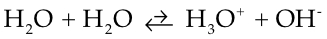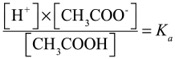## Blog Entries# Acids/Bases

The first thing you should know is the definitions of acid and base. It turns out there are several. For our purposes, an acid is a proton (H
+) donor and bases are proton acceptors. For a "reaction" to happen, you need both an acid and a base. However, water is often the other player in the reaction because it can be both an acid and a base (it's just equally bad at both). That property makes it ideal as the solvent for the reactions we will consider.

## pH.

You've heard the term; but, what does it mean? Consider the following reaction:The double arrow means it can go either direction and, in this case, favors the leftward direction.
One of the water molecules on the left is the proton donor (the acid) and the other is the proton acceptor (the base). Water is, as I said, equally bad at both. So, water can spontaneously give up a proton while another accepts it to become OH
- and H3O+, but does so to a very low extent. How low an extent? Well, we could express that in terms of what the concentration of OH- and H3O+ are in distilled water. The concentrations should be equal, since you produce them in a 1:1 ratio. By the way, we will abbreviate "Concentration of" by using square brackets. [H3O+] means "concentration of H3O+.
The concentration of H
+ (or H3O+) in distilled water is 10-7. There is a mathematical operation, taking the "p" of something is to take the negative log. So, the pH is 7 in distilled water. Note, I could talk about the pOH just as easily. It also would be 7 in distilled water, since the [OH- ] is also 10-7.
The sum of pH + pOH always will equal 14. As pH goes down (increasing [H
+]) the pOH goes up by the same amount (decreasing the [OH-])

For a strong acid (one that dissociates fully in water), the concentration of H+ can be taken directly from the concentration of the acid. So, if know the [HCl] is 0.025M. You can calculate the pH as follows:
All of the HCl will dissociate into H
+ and Cl-. So, the [H3O+] will be 0.025M. Take the log of that and you get -1.6. Convert the sign and you get pH=1.6.

## Conjugate acid/bases and Ka

What about weak acids? Well, weak acids, such as acetic acid (vinegar) don't dissociate fully.
The formula for acetic acid can be written:
CH
3COOH
That last "H" is the one that comes off as a proton. That's one reason we write it that way. In water
CH
3COOH +H2O CH3COO- + H3O+ The double arrow means the reaction can go both ways. So, reading the reaction left to right, CH3COOH is the proton donor (the acid) and H2O is the base (proton acceptor). Reading it right to left, the H3O+ is the acid and CH3COO- is the base. So, CH3COOH and CH3COO- are an acid and its conjugate base. Water and H3O+ are the base and conjugate acid of each other.

If I tell you that you have 0.025M CH
3COOH, you cannot conclude the pH is 1.6, as we did for the HCl, because "only a fraction" of the acid dissociates. The question is: "what is that fraction?" If I knew that, I could figure out the [H3O+] and therefore the pH.
Well, that fraction would be just the concentration of the dissociated ions divided by the amount that stays as the intact molecule. We call that fraction the K
a. K stands for "constant" (in German) and 'a' is for acid. It turns out that at any given temperature, an Acid has a measurable fraction that dissociates.
We write it like this:At 25℃, the Ka for Acetic acid is 1.8x10-5
Use that to determine what the pH of solution of 0.025M of acetic acid is. Hint, since the concentration of H+ and that of CH
3COO- are the same, you can treat the top of the fraction as "x2"

Back
MathJax Font